# Bipolar Transistor Biasing Circuits

## Discrete Semiconductor Devices and Circuits

• #### Question 1

 Don’t just sit there! Build something!!

Learning to mathematically analyze circuits requires much study and practice. Typically, students practice by working through lots of sample problems and checking their answers against those provided by the textbook or the instructor. While this is good, there is a much better way.

You will learn much more by actually building and analyzing real circuits, letting your test equipment provide the “answers” instead of a book or another person. For successful circuit-building exercises, follow these steps:

1. Carefully measure and record all component values prior to circuit construction, choosing resistor values high enough to make damage to any active components unlikely.
2. Draw the schematic diagram for the circuit to be analyzed.
3. Carefully build this circuit on a breadboard or other convenient medium.
4. Check the accuracy of the circuit’s construction, following each wire to each connection point, and verifying these elements one-by-one on the diagram.
5. Mathematically analyze the circuit, solving for all voltage and current values.
6. Carefully measure all voltages and currents, to verify the accuracy of your analysis.
7. If there are any substantial errors (greater than a few percent), carefully check your circuit’s construction against the diagram, then carefully re-calculate the values and re-measure.

When students are first learning about semiconductor devices, and are most likely to damage them by making improper connections in their circuits, I recommend they experiment with large, high-wattage components (1N4001 rectifying diodes, TO-220 or TO-3 case power transistors, etc.), and using dry-cell battery power sources rather than a benchtop power supply. This decreases the likelihood of component damage.

As usual, avoid very high and very low resistor values, to avoid measurement errors caused by meter “loading” (on the high end) and to avoid transistor burnout (on the low end). I recommend resistors between 1 kΩ and 100 kΩ.

One way you can save time and reduce the possibility of error is to begin with a very simple circuit and incrementally add components to increase its complexity after each analysis, rather than building a whole new circuit for each practice problem. Another time-saving technique is to re-use the same components in a variety of different circuit configurations. This way, you won’t have to measure any component’s value more than once.

• #### Question 2

Complete the table of output voltages for several given values of input voltage in this common-collector amplifier circuit. Assume that the transistor is a standard silicon NPN unit, with a nominal base-emitter junction forward voltage of 0.7 volts:Vin Vout 0.0 V 0.5 V 1.0 V 1.5 V 5.0 V 7.8 V

Based on the values you calculate, explain why the common-collector circuit configuration is often referred to as an emitter follower.

• #### Question 3

Describe what the output voltage of this transistor circuit will do (measured with reference to ground), if the potentiometer wiper begins at the full-down position (common with ground), and is slowly moved in the upward direction (closer to V):• #### Question 4

Describe what the output voltage of this transistor circuit will do (measured with reference to ground), if the input voltage ramps from 0 volts to -10 volts (measured with respect to ground):• #### Question 5

If we were to apply a sinusoidal AC signal to the input of this transistor amplifier circuit, the output would definitely not be sinusoidal: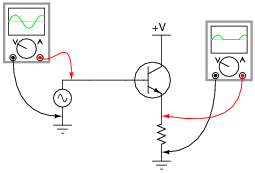It should be apparent that only portions of the input are being reproduced at the output of this circuit. The rest of the waveform seems to be “missing,” being replaced by a flat line. Explain why this transistor circuit is not able to amplify the entire waveform.

• #### Question 6

Class-A operation may be obtained from this simple transistor circuit if the input voltage (Vin) is “biased” with a series-connected DC voltage source: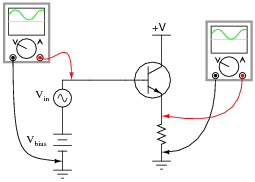First, define what “Class A” amplifier operation is. Then, explain why biasing is required for this transistor to achieve it.

• #### Question 7

Explain how the following bias networks function:Each one has the same basic purpose, but works in a different way to accomplish it. Describe the purpose of any biasing network in an AC signal amplifier, and comment on the different means of accomplishing this purpose employed by each of the three circuits.

Hint: imagine if the AC signal source in each circuit were turned off (replaced with a short). Explain how each biasing network maintains the transistor in a partially “on” state at all times even with no AC signal input.

• #### Question 8

Describe what the output voltage of this transistor circuit will do (measured with reference to ground), if the potentiometer wiper begins at the full-down position (common with ground), and is slowly moved in the upward direction (closer to V):• #### Question 9

If we were to apply a sinusoidal AC signal to the input of this transistor amplifier circuit, the output would definitely not be sinusoidal: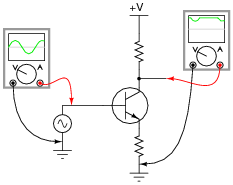It should be apparent that only portions of the input are being amplified in this circuit. The rest of the waveform seems to be “missing” in the output, being replaced by a flat line. Explain why this transistor circuit is not able to amplify the entire waveform.

• #### Question 10

Class-A operation may be obtained from this simple transistor circuit if the input voltage (Vin) is “biased” with a series-connected DC voltage source: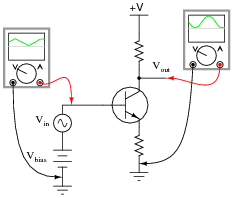First, define what “Class A” amplifier operation is. Then, explain why biasing is required for this transistor to achieve it.

• #### Question 11

Explain how the following bias networks function:Each one has the same basic purpose, but works in a different way to accomplish it. Describe the purpose of any biasing network in an AC signal amplifier, and comment on the different means of accomplishing this purpose employed by each of the three circuits.

• #### Question 12

A very common method of providing bias voltage for transistor amplifier circuits is with a voltage divider:However, if we were to directly connect a source of AC signal voltage to the junction between the two voltage divider resistors, the circuit would most likely function as if there were no voltage divider network in place at all:Instead, circuit designers usually place a coupling capacitor between the signal source and the voltage divider junction, like this:Explain why a coupling capacitor is necessary to allow the voltage divider to work in harmony with the AC signal source. Also, identify what factors would be relevant in deciding the size of this coupling capacitor.

• #### Question 13

Explain how it is possible for a fault in the biasing circuitry of a transistor amplifier to completely kill the (AC) output of that amplifier. How and why can a shift in DC bias voltage have an effect on the AC signal being amplified?

• #### Question 14

A student builds the following circuit and connects an oscilloscope to its output: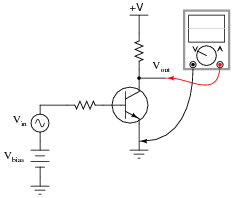The waveform shown on the oscilloscope display looks like this:Definitely not Class-A operation! Suspecting a problem with the input waveform, the student disconnects the oscilloscope probe from the amplifier output and moves it over to the amplifier input terminal. There, the following waveform is seen: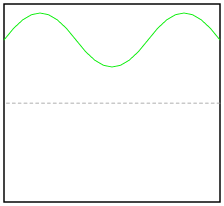How can this amplifier circuit be producing such a distorted output waveform with such a clean input waveform? Explain your answer.

• #### Question 15

Suppose you were building a Class-A transistor amplifier for audio frequency use, but did not have an oscilloscope available to check the output waveform for the presence of “clipping” caused by improper biasing. You do, however, have a pair of audio headphones you may use to listen to the signals.

Explain how you would use a pair of headphones to check for the presence of severe distortion in a waveform.

• #### Question 16

Calculate the approximate quiescent (DC) base current for this transistor circuit, assuming an AC input voltage of 0 volts, and a silicon transistor:• #### Question 17

Calculate the potentiometer wiper voltage (Vbias) required to maintain the transistor right at the threshold between cutoff and active mode. Then, calculate the input voltage required to drive the transistor right to the threshold between active mode and saturation. Assume ideal silicon transistor behavior, with a constant β of 100:• #### Question 18

When inserting a signal coupling capacitor into the bias network for this transistor amplifier, which way should the (polarized) capacitor go? (Hint: the AC signal source outputs pure AC, with a time-averaged DC value of 0 volts).Explain why the orientation of this capacitor matters, and what might happen if it is connected the wrong way.

• #### Question 19

Describe how proper biasing is accomplished in this headphone amplifier circuit (suitable for amplifying the audio output of a small radio):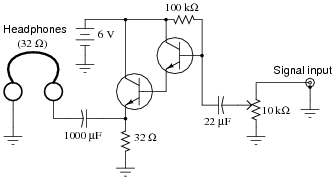Also, describe the functions of the 10 kΩ potentiometer and the 22 μF capacitor.

• #### Question 20

The following circuit is a three-channel audio mixer circuit, used to blend and amplify three different audio signals (coming from microphones or other signal sources):Suppose we measured a 9 kHz sinusoidal voltage of 0.5 volts (peak) at point Ä” in the diagram, using an oscilloscope. Determine the voltage at point “B” in the circuit, after this AC signal voltage “passes through” the voltage divider biasing network.

The voltage at point “B” will be a mix of AC and DC, so be sure to express both quantities! Ignore any “loading” effects of the transistor’s base current on the voltage divider.